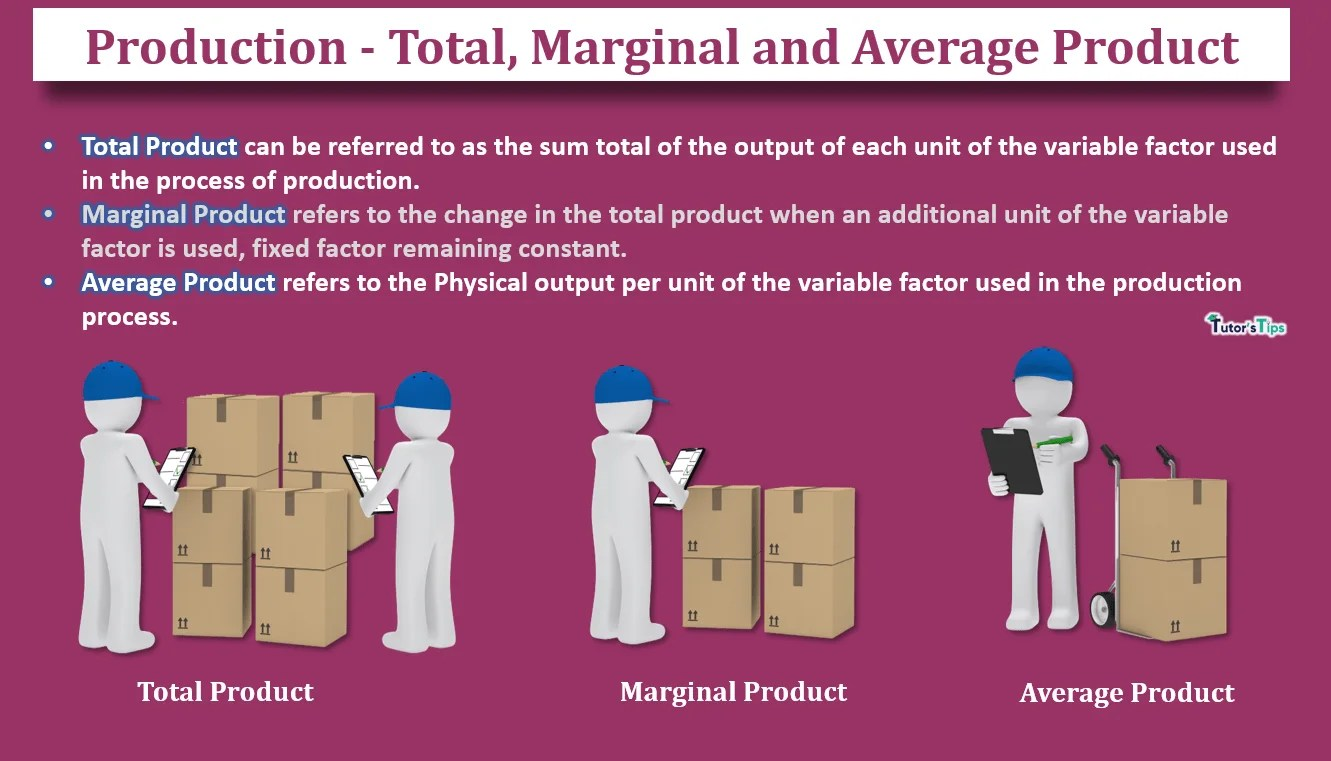# Production – Total, Marginal and Average ProductThe total product, marginal product and average product are vital aspects of production or output.

## What is Production :

Production refers to the amount of output using variable and fixed factors of production as inputs. In other words, it is the quantity of goods and services produced by a firm or industry whether to consume or to use in further processing.

The production can be classified as:

1. Total Product
2. Marginal Product
3. Average Product

## Total Product :

Total product is the sum total of output produced by all the units of variable inputs with a constant amount of fixed input used in the process of production. It is also known as Total Physical Product or TPP or TP.

Suppose, using Land as fixed factor and labour as a variable factor, the producer is able to produce :

 Labour used as a variable input (in units) Product/ output (in units) 1 5 2 10 3 15 4 10 5 5 Total Product= 5+10+15+10+5 = 45 units

Here, with one unit of labour, the producer is able to produce 5 units of a commodity. But, as he increases the use of labour to 2 nits, the output also increases to 10 units. Likewise, 3 units of labour increase the output to 15 units, which is maximum. As, after this, the increase in employment of labour decreases the output to 10 units and then to 5 units. Thus, the Total Product can be referred to as the sum total of the output of each unit of the variable factor used in the process of production. Hence, it is also known as Total Return of the Variable Factor.

## Marginal Product :

Marginal Product refers to the change in the total product when one more unit of the variable factor is used with constant use of fixed factor. It is also called ass Marginal Physical Product or MPP or MP.

It can be calculated as:

MPn = TPn – TPn-1

Here,

MPdenotes the Marginal Product from using nth unit of the variable factor

TPdenotes Total Product from nth unit of the variable factor

TPn-1 denotes Total Product from (n-1)th unit of the variable factor

Suppose, using Land as fixed factor and labour as a variable factor, the producer is able to produce :

 Labour used as a variable input (in units) Total Product/ output (in units) Marginal Product (in units) 1 5 5-0 =5 2 8 8-5=3 3 15 15-8=7 4 12 12-15=-3 5 10 10-12 =-2 Total Product= 5+10+15+10+5 =45 units

Here, with one unit of labour, the producer is able to produce 5 units of a commodity. But, as he increases the use of labour to 2 units, the output also increases to 10 units resulting in a marginal product as 5 units. Likewise, 3 units of labour increase the output to 15 units, which is maximum with the maximum marginal product as 7 units. As, after this, the increase in employment of labour decreases the output to 10 units and then to 5 units leading to the negative marginal product as -3 and -2 respectively. Thus, the Marginal Product refers to the change in the total product when an additional unit of the variable factor is used, fixed factor remaining constant.

Therefore, the Total Product is the total of Marginal Product corresponding to each unit of the variable factor.

## Average Product :

It refers to the output per unit of the variable factor. It can be calculated as :

AP = TP/ L

Here,

AP denotes Average Product

TP denotes Total Product

L denotes the units of the variable factor

For Example, If the total product is 50 units when 10 units of labour are used, then:

AP = 50/10 = 5 units of output

Suppose, using Land as fixed factor and labour as a variable factor, the producer is able to produce :

 Labour used as a variable input (in units) Total Product/ output (in units) Average Product (in units) 1 5 5/1 =5 2 8 8/2=4 3 9 9/3=3 4 8 8/4=2 5 5 5/5=1 Total Product= 5+10+15+10+5 =45 units

Here, with one unit of labour, the producer is able to produce 5 units of a commodity with an average product of 5 units. But, as he increases the use of labour to 2 units, the output also increases to 10 units resulting to an average product as 4 units. Likewise, 3 units of labour increase the output to 15 units, which is maximum with the average product as 3 units. As, after this, the increase in employment of labour decreases the output to 10 units and then to 5 units leading to the marginal product as 2 and 1 unit respectively.

Thus, the Average Product refers to the Physical output per unit of the variable factor used in the production process.

Comment if you have any question.

References:

Introductory Microeconomics – Class 11 – CBSE (2020-21)